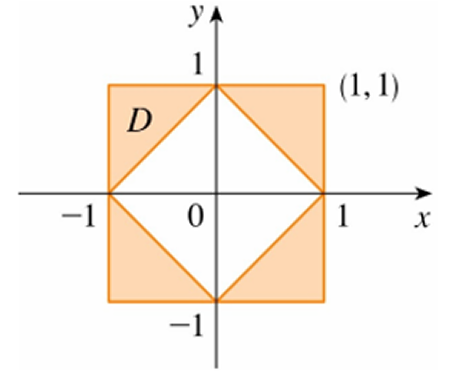# Set up, but do not compute the iterated integral(s) f(x,y)dA, where D is the shaded region shown,...

## Question:

Set up, but do not compute the iterated integral(s) {eq}\int\int_{D}f(x,y)dA {/eq}, where D is the shaded region shown, by separating the region as appropriate:## Double Integrals

The integral of {eq}\displaystyle f(x,y) {/eq} over a region {eq}\displaystyle D {/eq} is

{eq}\displaystyle \iint_{D}f(x,y)dA {/eq} and evaluated iteratively as {eq}\displaystyle \int_a^b\int_{u(y)}^{v(y)}f(x,y) dxdy, {/eq}

if the region is described as an horizontally-simple region, {eq}\displaystyle D=\{(x,y)|\, a\leq y\leq b, u(y)\leq x\leq v(y)\}. {/eq}

If the integrand function is not easy to integrate with respect to {eq}\displaystyle x, {/eq} then we need to change the order of integration, by describing the region as:

{eq}\displaystyle \displaystyle D=\{(x,y)|\, c\leq x\leq d, w(x)\leq y\leq h(x)\} {/eq}

and the integral is {eq}\displaystyle \int_c^d\int_{w(x)}^{h(x)}f(x,y) dydx. {/eq}

To write the double integral of a function {eq}\displaystyle f(x,y) {/eq} over the region {eq}\displaystyle D {/eq} shown in the picture we need to describe the four corners as below.

The corner in quadrant I, is described vertically simple as being bounded below by the line {eq}\displaystyle x+y=1 \text{ and above by }y=1, \text{ for } 0\leq x\leq 1, {/eq} or

{eq}\displaystyle \begin{align} D_I=\{ (x,y)| \ 0\leq x\leq 1, 1-x\leq y\leq 1\} \end{align} {/eq}

The corner in quadrant II, is described vertically simple as being bounded below by the line {eq}\displaystyle -x+y=1 \text{ and above by }y=1, \text{ for } -1\leq x\leq 0, {/eq} or

{eq}\displaystyle \begin{align} D_{II}=\{ (x,y)| \ -1\leq x\leq 0, 1+x\leq y\leq 1\} \end{align} {/eq}

The corner in quadrant III, is described vertically simple as being bounded below by the line {eq}\displaystyle y=-1 \text{ and above by }-x-y=1, \text{ for } -1\leq x\leq 0, {/eq} or

{eq}\displaystyle \begin{align} D_{III}=\{ (x,y)| \ -1\leq x\leq 0, -1\leq y\leq -x-1\} \end{align} {/eq}

And the corner in quadrant IV, is described vertically simple as being bounded below by the line {eq}\displaystyle y=-1 \text{ and above by }x-y=1, \text{ for } 0\leq x\leq 1, {/eq} or

{eq}\displaystyle \begin{align} D_{IV}=\{ (x,y)| \ 0\leq x\leq 1, -1\leq y\leq x-1\}. \end{align} {/eq}

Therefore the double integral is written as the sum of the following four iterated integrals

The corner in quadrant III, is described vertically simple as being bounded below by the line {eq}\displaystyle y=-1 \text{ and above by }-x-y=1, \text{ for } -1\leq x\leq 0, {/eq} or

{eq}\displaystyle \begin{align} \iint_{D} f(x,y)\ dA&= \iint_{D_{I}} f(x,y)\ dA+ \iint_{D_{II}} f(x,y)\ dA+ \iint_{D_{III}} f(x,y)\ dA+ \iint_{D_{IV}} f(x,y)\ dA\\ &= \boxed{\int _0^1\int_{1-x}^1 f(x,y)\ dydx+\int _{-1}^0\int_{1+x}^1 f(x,y)\ dydx+\int _{-1}^0\int_{-1}^{-x-1} f(x,y)\ dydx+ \int _0^1\int_{-1}^{x-1} f(x,y)\ dydx}. \end{align} {/eq}